# comm.MemorylessNonlinearity

Apply memoryless nonlinearity to complex baseband signal

## Description

The `comm.MemorylessNonlinearity` System object™ applies memoryless nonlinear impairments to a baseband signal. Use this System object to model memoryless nonlinear impairments caused by signal amplification in a radio frequency (RF) transmitter or receiver. For more information, see Memoryless Nonlinear Impairments.

To apply memoryless nonlinear impairments to a complex baseband signal:

1. Create the `comm.MemorylessNonlinearity` object and set its properties.

2. Call the object with arguments, as if it were a function.

## Creation

### Syntax

``mnl = comm.MemorylessNonlinearity``
``mnl = comm.MemorylessNonlinearity(Name,Value)``

### Description

````mnl = comm.MemorylessNonlinearity` creates a memoryless nonlinearity System object that models RF nonlinear impairments.```

example

````mnl = comm.MemorylessNonlinearity(Name,Value)` specifies properties using one or more name-value pair arguments. Enclose each property name in quotes. For example, `'Method','Saleh model'` sets the modeling method to the Saleh method.```

## Properties

expand all

Unless otherwise indicated, properties are nontunable, which means you cannot change their values after calling the object. Objects lock when you call them, and the `release` function unlocks them.

If a property is tunable, you can change its value at any time.

Nonlinearity modeling method, specified as `'Cubic polynomial'`, `'Hyperbolic tangent'`, `'Saleh model'`, `'Ghorbani model'`, `'Modified Rapp model'`, or `'Lookup table'`. For more information, see Memoryless Nonlinear Impairments.

Data Types: `char` | `string`

Input signal scaling factor in decibels, specified as a scalar. This property scales the power gain of the input signal.

Tunable: Yes

#### Dependencies

To enable this property, set the `Method` property to `'Saleh model'` or ```'Ghorbani model'```.

Data Types: `double`

Linear gain in decibels, specified as a scalar. This property scales the power gain of the output signal.

Tunable: Yes

#### Dependencies

To enable this property, set the `Method` property to `'Cubic polynomial'`, ```'Hyperbolic tangent'```, or `'Modified Rapp model'`.

Data Types: `double`

Third-order nonlinearity specification for cubic polynomial, specified as `'IIP3'`, `'OIP3'`, `'IP1dB'`, `'OP1dB'`, `'IPsat'`, or `'OPsat'`. For more information, see Cubic Polynomial Third-Order Coefficient.

#### Dependencies

To enable this property, set the `Method` property to `'Cubic polynomial'`.

Data Types: `char` | `string`

Third-order input intercept point in dBm, specified as a scalar.

Tunable: Yes

#### Dependencies

To enable this property, set the `Method` property to `'Cubic polynomial'` or ```'Hyperbolic tangent'```.

Data Types: `double`

Third-order output intercept point in dBm, specified as a scalar.

Tunable: Yes

#### Dependencies

To enable this property, set the `Method` property to `'Cubic polynomial'` and the `TOISpecification` property to `'OIP3'`.

Data Types: `double`

One dB input compression point in dBm, specified as a scalar.

Tunable: Yes

#### Dependencies

To enable this property, set the `Method` property to `'Cubic polynomial'` and the `TOISpecification` property to `'IP1dB'`.

Data Types: `double`

One dB output compression point in dBm, specified as a scalar.

Tunable: Yes

#### Dependencies

To enable this property, set the `Method` property to `'Cubic polynomial'` and the `TOISpecification` property to `'OP1dB'`.

Data Types: `double`

Input saturation point in dBm, specified as a scalar.

Tunable: Yes

#### Dependencies

To enable this property, set the `Method` property to `'Cubic polynomial'` and the `TOISpecification` property to `'IPsat'`.

Data Types: `double`

Output saturation point in dBm, specified as a scalar.

Tunable: Yes

#### Dependencies

To enable this property, set the `Method` property to `'Cubic polynomial'` and the `TOISpecification` property to `'OPsat'`.

Data Types: `double`

Linear AM/PM conversion factor in degrees per decibel, specified as a scalar. For more information, see Cubic Polynomial and Hyperbolic Tangent Model Methods.

Tunable: Yes

#### Dependencies

To enable this property, set the `Method` property to `'Cubic polynomial'` or ```'Hyperbolic tangent'```.

Data Types: `double`

AM/AM parameters used to compute the amplitude gain for an input signal, specified as a row vector.

• When the `Method` property is set to `'Saleh model'`, this property must be a two-element vector that specifies alpha and beta values. In this case, the default value is `[2.1587 1.1517]`.

• When the `Method` property is set to `'Ghorbani model'`, this property must be a four-element vector that specifies x1, x2, x3, and x4 values. In this case, the default value is `[8.1081 1.5413 6.5202 -0.0718]`.

Tunable: Yes

#### Dependencies

To enable this property, set the `Method` property is set to `'Saleh model'` or ```'Ghorbani model'```.

Data Types: `double`

AM/PM parameters used to compute the phase change for an input signal, specified as a row vector.

• When the `Method` property is set to `'Saleh model'`, this property must be a two-element vector that specifies alpha and beta values. In this case, the default value is `[4.0033 9.1040]`.

• When the `Method` property is set to `'Ghorbani model'`, this property must be a four-element vector that specifies y1, y2, y3, and y4 values. In this case, the default value is `[4.6645 2.0965 10.88 -0.003]`

Tunable: Yes

#### Dependencies

To enable this property, set the `Method` property is set to `'Saleh model'` or ```'Ghorbani model'```.

Data Types: `double`

Input power lower limit in dBm, specified as a scalar less than the `PowerUpperLimit` property value. The AM/PM conversion scales linearly for input power values in the range [`PowerLowerLimit`, `PowerUpperLimit`]. If the input signal power is below the input power lower limit, the phase shift resulting from AM/PM conversion is zero. For more information, see Cubic Polynomial and Hyperbolic Tangent Model Methods.

Tunable: Yes

#### Dependencies

To enable this property, set the `Method` property is set to `'Cubic polynomial'` or ```'Hyperbolic tangent'```.

Data Types: `double`

Input power upper limit in dBm, specified as a scalar greater than `PowerLowerLimit`. The AM/PM conversion scales linearly for input power values in the range [`PowerLowerLimit`, `PowerUpperLimit`]. If the input signal power is above the input power upper limit, the phase shift resulting from AM/PM conversion is constant. For more information, see Cubic Polynomial and Hyperbolic Tangent Model Methods.

Tunable: Yes

#### Dependencies

To enable this property, set the `Method` property is set to `'Cubic polynomial'` or ```'Hyperbolic tangent'```.

Data Types: `double`

Output signal scaling factor in decibels, specified as a scalar. This property scales the power gain of the output signal.

Tunable: Yes

#### Dependencies

To enable this property, set the `Method` property is set to `'Saleh model'` or ```'Ghorbani model'```.

Data Types: `double`

Amplitude smoothness factor, specified as a scalar. For more information, see Modified Rapp Model Method.

Tunable: Yes

#### Dependencies

To enable this property, set the `Method` property is set to `'Modified Rapp model'`.

Data Types: `double`

Phase gain for modified Rapp model in radians, specified as a scalar. A value of `-0.45` is typical. For more information, see Modified Rapp Model Method.

Tunable: Yes

#### Dependencies

To enable this property, set the `Method` property is set to `'Modified Rapp model'`.

Data Types: `double`

Phase saturation for modified Rapp model in radians, specified as a positive scalar. For more information, see Modified Rapp Model Method.

Tunable: Yes

#### Dependencies

To enable this property, set the `Method` property is set to `'Modified Rapp model'`.

Data Types: `double`

Phase smoothness for modified Rapp model in radians, specified as a positive scalar. For more information, see Modified Rapp Model Method.

Tunable: Yes

#### Dependencies

To enable this property, set the `Method` property is set to `'Modified Rapp model'`.

Data Types: `double`

Output saturation level, specified as a scalar. For more information, see Modified Rapp Model Method.

Tunable: Yes

#### Dependencies

To enable this property, set the `Method` property is set to `'Modified Rapp model'`.

Data Types: `double`

Amplifier characteristics lookup table, specified as an N-by-3 matrix of measured power amplifier (PA) characteristics. Each row is of the form [Pin, Pout, ΔΦ]. Pin specifies the input signal in dBm, Pout specifies the output signal in dBm, and ΔΦ specifies the output phase shift in degrees. The default value is ```[-25, 5.16, -0.25; -20, 10.11, -0.47; -15, 15.11, -0.68; -10, 20.05, -0.89; -5, 24.79, -1.22; 0, 27.64, 5.59; 5, 28.49, 12.03]```.

The measured PA characteristics defined by this property are used to compute the AM/AM (in dBm/dBm) and AM/PM (in deg/dBm) nonlinear impairment characteristics.

Note

To determine appropriate Pout and ΔΦ values for any Pin values outside the range of values specified in the `Table` property, the System object applies linear extrapolation from the first two or last two [Pin, Pout, ΔΦ] rows of `Table`.

Tunable: Yes

#### Dependencies

To enable this property, set the `Method` property to `'Lookup table'`.

Data Types: `double`

Reference impedance in ohms, specified as a positive scalar. This value is used to convert voltage values to power values.

Tunable: Yes

Data Types: `double`

## Usage

### Syntax

``outsig = mnl(insig)``

### Description

example

````outsig = mnl(insig)` applies memoryless nonlinear impairments to the input RF baseband signal.```

### Input Arguments

expand all

Input RF baseband signal, specified as a scalar, column vector, or matrix.

Data Types: `double`

### Output Arguments

expand all

Output RF baseband signal, returned as a scalar, column vector, or matrix. The output is of the same data type as the input.

## Object Functions

To use an object function, specify the System object as the first input argument. For example, to release system resources of a System object named `obj`, use this syntax:

`release(obj)`

expand all

 `release` Release resources and allow changes to System object property values and input characteristics `clone` Create duplicate System object `isLocked` Determine if System object is in use ```plot (memorylessnonlinearity)``` Plot nonlinearity AM/AM and AM/PM characteristics
 `step` Run System object algorithm `release` Release resources and allow changes to System object property values and input characteristics `reset` Reset internal states of System object

## Examples

collapse all

Apply cubic polynomial nonlinearity to two 16-QAM signals. The first input signal power level is in the linear region of the amplifier power characteristic curve. The second input signal power level is in the nonlinear region of the amplifier power characteristic curve. Show the amplifier power characteristic curve and the constellation diagram for the amplified 16-QAM signals.

Initialize Simulation

Initialize variables for simulation and create System objects for a memoryless nonlinearity amplifier impairment and a constellation diagram. So that the constellation shows power compression only (and no phase rotation), configure the memoryless nonlinearity amplifier impairment with AM-PM distortion set to zero.

```M = 16; % Modulation order sps = 4; % Samples per symbol pindBm = [12 25]; % Input power gain = 10; % Amplifier gain amplifier = comm.MemorylessNonlinearity("Method","Cubic polynomial", ... "LinearGain",gain,"AMPMConversion",0,"ReferenceImpedance",50); refConst = qammod([0:M-1],M); axisLimits = [-gain gain]; constdiag = comm.ConstellationDiagram("NumInputPorts",2, ... "ChannelNames",["Linear" "Nonlinear"],"ShowLegend",true, ... "ReferenceConstellation",refConst, ... "XLimits",axisLimits,"YLimits",axisLimits);```

Amplify and Plot Signal

Apply 16-QAM to an input signal of random data. Amplify the signal and use the `plot` function of the `comm.MemorylessNonlinearity` System object to show the output power and phase response curves. The first input signal power level is 12 dBm and is in the linear region of the amplifier power characteristic curve. The second input signal power level is 25 dBm and is in the nonlinear region of the amplifier power characteristic curve.

```pin = 10.^((pindBm-30)/10); % Convert dBm to linear Watts data = randi([0 M-1],1000,1); modOut = qammod(data,M,"UnitAveragePower",true)*sqrt(pin*amplifier.ReferenceImpedance); ampOut = amplifier(modOut); plot(amplifier);```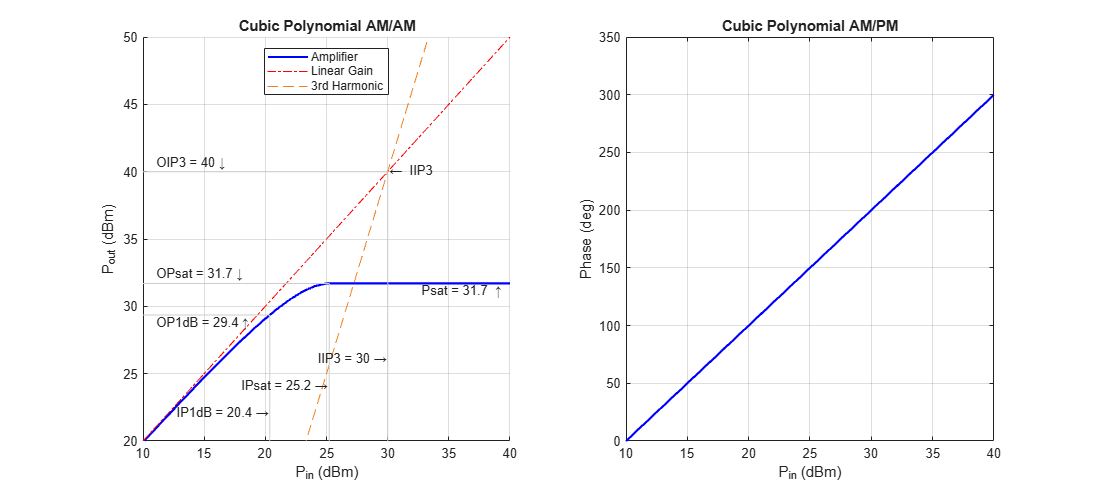Add AWGN to the two amplified signals and show the constellation diagram for the signals.

```snr = 25; noisyLinOut = awgn(ampOut(:,1),snr,"measured"); noisyNonLinOut = awgn(ampOut(:,2),snr,"measured"); constdiag(noisyLinOut,noisyNonLinOut);```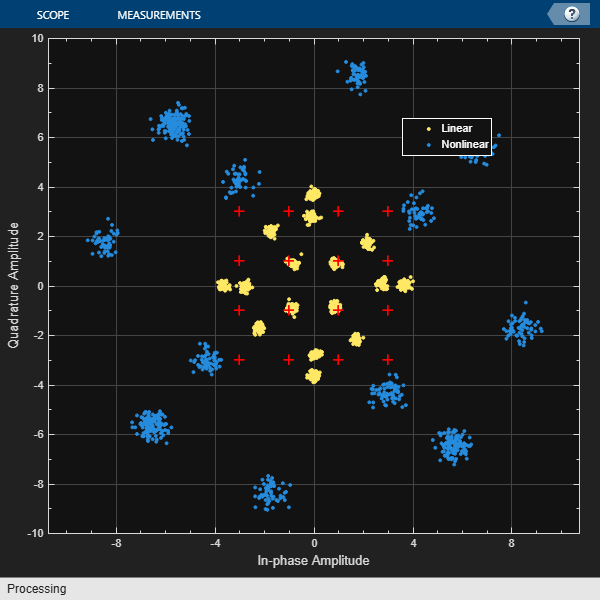Generate 16-QAM data with an average power of 10 mW and a reference impedance of 1 ohm. Pass the data through a nonlinear power amplifier (PA).

```M = 16; data = randi([0 (M - 1)]',1000,1); avgPow = 1e-2; minD = avgPow2MinD(avgPow,M);```

Create a memoryless nonlinearity System object, specifying the Saleh model method.

`saleh = comm.MemorylessNonlinearity('Method','Saleh model');`

Generate modulated symbols and pass them through the PA nonlinearity model.

```modData = (minD/2).*qammod(data,M); y = saleh(modData);```

Generate a scatter plot of the results.

`scatterplot(y)`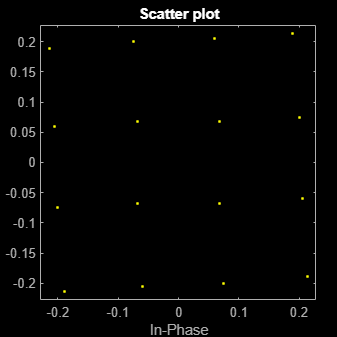Average power normalization of input signal.

```function minD = avgPow2MinD(avgPow,M) % Average power to minimum distance nBits = log2(M); if (mod(nBits,2)==0) % Square QAM sf = (M - 1)/6; else % Cross QAM if (nBits>4) sf = ((31*M/32) - 1)/6; else sf = ((5*M/4) - 1)/6; end end minD = sqrt(avgPow/sf); end```

Plot the gain compression of a nonlinear amplifier for a 16-QAM signal.

Specify the modulation order and samples per symbol parameters.

```M = 16; sps = 4;```

Model a nonlinear amplifier, by creating a memoryless nonlinearity System object with a 30 dB third-order input intercept point. Create a raised cosine transmit filter System object.

```amplifier = comm.MemorylessNonlinearity('IIP3',30); txfilter = comm.RaisedCosineTransmitFilter( ... 'RolloffFactor',0.3,'FilterSpanInSymbols',6, ... 'OutputSamplesPerSymbol',sps,'Gain',sqrt(sps));```

Specify the input power in dBm and a reference impedance of 1 ohm. Convert the input power to W and initialize the gain vector.

```pindBm = -5:25; pin = 10.^((pindBm-30)/10); gain = zeros(length(pindBm),1);```

Execute the main processing loop, which includes these steps.

• Generate random data symbols.

• Modulate the data symbols and adjust the average power of the signal.

• Filter the modulated signal.

• Amplify the signal.

• Measure the gain.

```for k = 1:length(pin) data = randi([0 (M - 1)],1000,1); modSig = qammod(data,M,'UnitAveragePower',true)*sqrt(pin(k)); filtSig = txfilter(modSig); ampSig = amplifier(filtSig); gain(k) = 10*log10(mean(abs(ampSig).^2) / mean(abs(filtSig).^2)); end```

Plot the amplifier gain as a function of the input signal power. The 1 dB gain compression point occurs for an input power of 18.5 dBm. To increase the point at which a 1 dB compression is observed, increase the third-order intercept point, `amplifier.IIP3`.

```arrayplot = dsp.ArrayPlot('PlotType','Line','XLabel','Power In (dBm)', ... 'XOffset',-5,'YLimits',[-5 5]); arrayplot(gain)```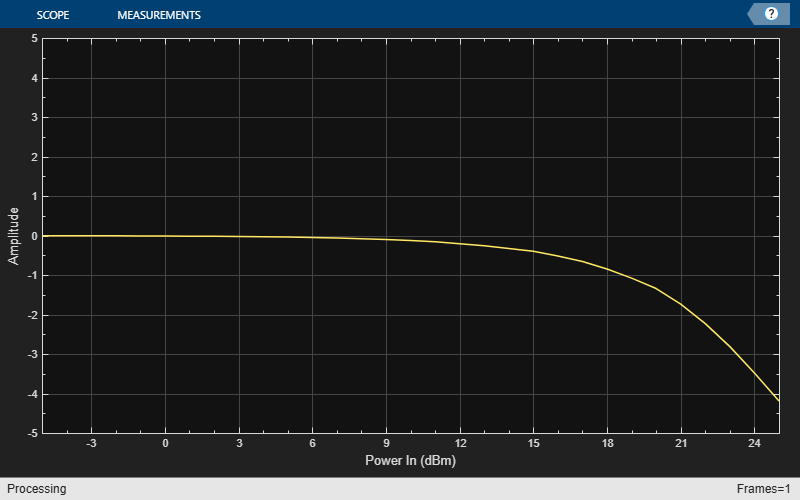Apply nonlinear power amplifier (PA) characteristics with 50 $\Omega$ impedance to a 16-QAM signal. Load PA characteristics by setting the `Method` property to `'Lookup table'`. The `pa_performance_characteristics` helper function outputs the amplifier performance characteristics lookup table.

Define parameters for the modulation order, samples per symbol, and input power. Create random data.

```M = 16; % Modulation order sps = 4; % Samples per symbol pindBm = -8; % Input power pin = 10.^((pindBm-30)/10); % power in Watts data = randi([0 (M - 1)],1000,1); refdata = 0:M-1; refconst = qammod(refdata,M,'UnitAveragePower',true); paChar = pa_performance_characteristics();```

Create a memoryless nonlinearity System object, a transmit filter System object, and a constellation diagram System object. The default lookup table values are used for the memoryless nonlinearity System object.

```amplifier = comm.MemorylessNonlinearity('Method','Lookup table','Table',paChar,'ReferenceImpedance',50); txfilter = comm.RaisedCosineTransmitFilter('RolloffFactor',0.3, ... 'FilterSpanInSymbols',6,'OutputSamplesPerSymbol',sps,'Gain',sqrt(sps)); constellation = comm.ConstellationDiagram('SamplesPerSymbol',4, ... 'Title','Amplified/Distorted Signal','NumInputPorts',2, ... 'ReferenceConstellation', refconst,'ShowLegend',true, ... 'ChannelNames',{'Filtered signal','Amplified signal'});```

Modulate the random data. Filter and apply the nonlinear amplifier characteristics to the modulation symbols.

```modSig = qammod(data,M,'UnitAveragePower',true)*sqrt(pin * amplifier.ReferenceImpedance); filtSig = txfilter(modSig); ampSig = amplifier(filtSig);```

Compute input and output signal levels and the phase shift.

```pSig = abs(ampSig).^2 / amplifier.ReferenceImpedance; poutdBm = 10 * log10(pSig) + 30; pfiltSig = abs(filtSig).^2 / amplifier.ReferenceImpedance; simulated_pindBm = 10 * log10(pfiltSig) + 30; phase = rad2deg(angle(ampSig.*conj(filtSig)));```

Plot AM/AM characteristics, AM/PM characteristics, and the constellation results.

```figure set(gcf,'units','normalized','position',[.25 1/3 .5 1/3]) subplot(1,2,1) plot(simulated_pindBm,poutdBm,'.'); hold on plot(amplifier.Table(:,1),amplifier.Table(:,2),'.','Markersize',15); xlabel('Input Power (dBm)') ylabel('Output Power (dBm)'); grid on; title('AM/AM Characteristics'); leglabel = {'Simulated results','Measurement'}; legend (leglabel,'Location','south'); subplot(1,2,2) plot(simulated_pindBm,phase,'.'); hold on plot(amplifier.Table(:,1),amplifier.Table(:,3),'.','Markersize',15); legend (leglabel,'Location','south'); xlabel('Input Power (dBm)'); ylabel('Output Phase Shift (degrees)'); grid on; title('AM/PM Characteristics');```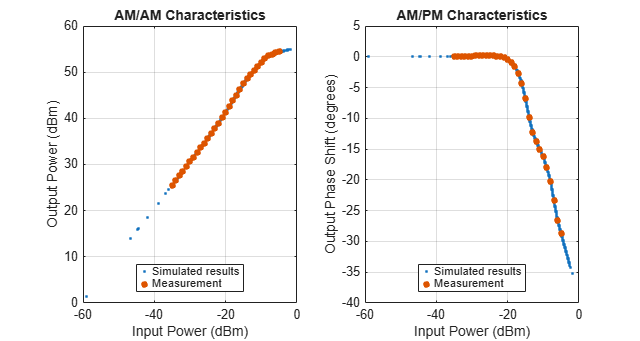For the purpose of constellation comparison, normalize the amplified signal and the filtered signal. Generate a constellation diagram of the filtered signal and amplified signal. The nonlinear amplifier characteristics cause compression of the amplified signal constellation compared to the filtered constellation.

```filtSig = filtSig/mean(abs(filtSig)); % Normalized filtered signal ampSig = ampSig/mean(abs(ampSig)); % Normalized amplified signal constellation(filtSig,ampSig)```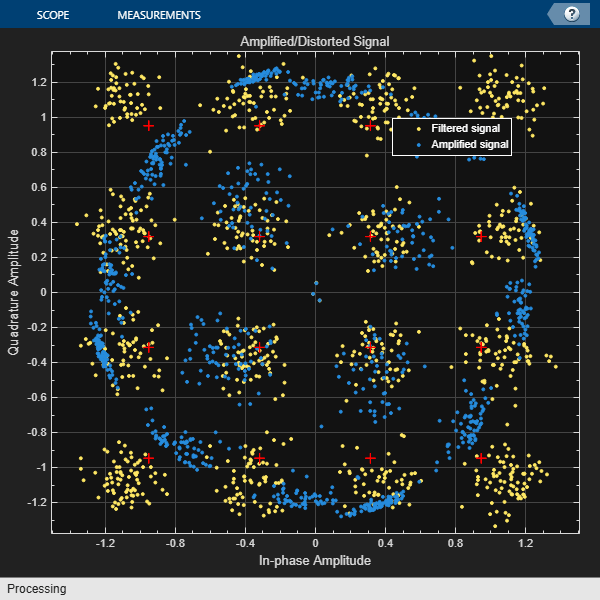Helper Function

`function paChar = pa_performance_characteristics()`

The operating specification for the LDMOS-based Doherty amplifier are:

• A frequency of 2110 MHz

• A peak power of 300 W

• A small signal gain of 61 dB

Each row in `HAV08_Table` specifies Pin (dBm), gain (dB), phase shift (degrees) as derived from figure 4 of Hammi, Oualid, et al. "Power amplifiers' model assessment and memory effects intensity quantification using memoryless post-compensation technique." IEEE Transactions on Microwave Theory and Techniques 56.12 (2008): 3170-3179.

```HAV08_Table =... [-35,60.53,0.01; -34,60.53,0.01; -33,60.53,0.08; -32,60.54,0.08; -31,60.55,0.1; -30,60.56,0.08; -29,60.57,0.14; -28,60.59,0.19; -27,60.6,0.23; -26,60.64,0.21; -25,60.69,0.28; -24,60.76,0.21; -23,60.85,0.12; -22,60.97,0.08; -21,61.12,-0.13; -20,61.31,-0.44; -19,61.52,-0.94; -18,61.76,-1.59; -17,62.01,-2.73; -16,62.25,-4.31; -15,62.47,-6.85; -14,62.56,-9.82; -13,62.47,-12.29; -12,62.31,-13.82; -11,62.2,-15.03; -10,62.15,-16.27; -9,62,-18.05; -8,61.53,-20.21; -7,60.93,-23.38; -6,60.2,-26.64; -5,59.38,-28.75];```

Convert the second column of the HAV08_Table from gain to Pout for use by the memoryless nonlinearity System object.

```paChar = HAV08_Table; paChar(:,2) = paChar(:,1) + paChar(:,2); end```

expand all

 Saleh, A.A.M. “Frequency-Independent and Frequency-Dependent Nonlinear Models of TWT Amplifiers.” IEEE Transactions on Communications 29, no. 11 (November 1981): 1715–20. https://doi.org/10.1109/TCOM.1981.1094911.

 Ghorbani, A., and M. Sheikhan. "The Effect of Solid State Power Amplifiers (SSPAs) Nonlinearities on MPSK and M-QAM Signal Transmission." In 1991 Sixth International Conference on Digital Processing of Signals in Communications, 193–97, 1991.

 Rapp, Ch. "Effects of HPA-Nonlinearity on a 4-DPSK/OFDM-Signal for a Digital Sound Broadcasting System." In Proceedings Second European Conf. on Sat. Comm. (ESA SP-332), 179–84. Liege, Belgium, 1991. https://elib.dlr.de/33776/.

 Choi, C., et.al. "RF impairment models for 60 GHz-band SYS/PHY simulation." IEEE 802.15-06-0477-01-003c. November 2006.

 Perahia, E. "TGad Evaluation Methodology." IEEE 802.11-09/0296r16. January 20, 2010. https://mentor.ieee.org/802.11/dcn/09/11-09-0296-16-00ad-evaluation-methodology.doc.

 Kundert, Ken. "Accurate and Rapid Measurement of IP2 and IP3." The Designer Guide Community. May 22, 2002.